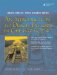# Pointer Arithmetic

The result of applying the operators +, -, ++, or -- to a pointer depends on the type of object pointed to. When an arithmetic operator is applied to a pointer p of type T*, p is assumed to point to an element of an array of objects of type T.

• p+1 points to the next element of the array.
• p-1 points to the preceding element of the array.
• In general, the address p+k is k*sizeof(T) bytes larger than the address p.

Subtraction of pointers is defined only when both pointers point to elements of the same array. In this case the difference is an int equal to the number of array elements between the two elements.

The results of pointer arithmetic are undefined outside the context of an array. It is the responsibility of the programmer to ensure that pointer arithmetic is used appropriately.

Example 22.4. src/arrays/pointerArith.cpp

 ```[ . . . . ] int main() { using namespace std; int y[] = {3, 6, 9}; int x = 23; int* px; px = &y; <-- 1 px = y; <-- 2 cout << "What's next: " << *++px << endl; cout << "What's next: " << *++px << endl; cout << "What's next: " << *++px << endl; cout << "What's next: " << *++px << endl; return 0; } ```   (1)The & is redundant here. (2)y, or any array name, is an "alias" for a pointer to the first element in the array.

If we ran the above example, we might see something like this:

 In general, accessing memory beyond the boundary of an array is nonportable, and since that is what we are doing (on purpose), the results will also be nonportable.

```What's next: 6
What's next: 9
What's next: 23
What's next: -1073751080
```

Notice that neither the compiler nor the run-time system reported an error message. C++ happily reads from arbitrary memory addresses and reports them in the type of your choice, giving the C++ developer great power and many opportunities to make great errors.

### Arrays, Functions, and Return ValuesAn Introduction to Design Patterns in C++ with Qt 4
ISBN: 0131879057
EAN: 2147483647
Year: 2004
Pages: 268